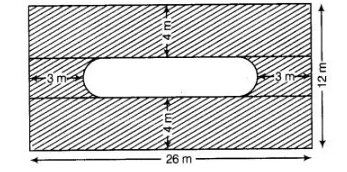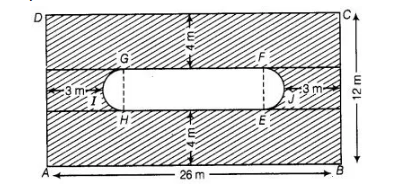# Find the area of the shaded region in figure.

Question:

Find the area of the shaded region in figure.Solution:

join GH and FEHere, breadth of the rectangle $B C=12 \mathrm{~m}$

$\therefore$ Breadth of the inner rectangle $E F G H=12-(4+4)=4 \mathrm{~cm}$

which is equal to the diameter of the semi-circle $E J F, d=4 \mathrm{~m}$

$\therefore \quad$ Radius of semi-circle $E J F, r=2 \mathrm{~m}$

$\therefore \quad$ Length of inner rectangle $E F G H=26-(5+5)=16 \mathrm{~m}$

$\therefore$ Area of two semi-circles EJF and $H / G=2\left(\frac{\pi r^{2}}{2}\right)=2 \times \pi \frac{(2)^{2}}{2}=4 \pi \mathrm{m}$

Now, $\quad$ area of inner rectangle $E F G H=E H \times F G=16 \times 4=64 \mathrm{~m}^{2}$

and area of outer rectangle $A B C D=26 \times 12=312 \mathrm{~m}^{2}$

$\therefore \quad$ Area of shaded region $=$ Area of outer rectangle $-($ Area of two semi-circles

+ Area of inner rectangle)

$=312-(64+4 \pi)=(248-4 \pi) \mathrm{m}^{2}$